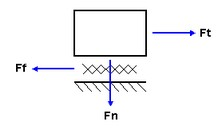﻿ 20-sim webhelp > Modeling Tutorial > Friction > Normal Force

# Normal Force

Assume a mass is dragged over a surface and experiences friction.A tension force Ft has to be applied to overcome the amount of friction force Ff. The friction force will depend on the applied normal force as:

Ft = Fn * f(x,v,...);

where f is the friction function which depends on the displacement x, velocity v etc.

In most cases a normal force is available (disk breaks, surface friction, clutches etc.). Therefore all models in the 20-sim friction library are described with a normal force input. For those models that do not experience a normal force (bearings), a unity normal force Fn = 1 [N] is applied.